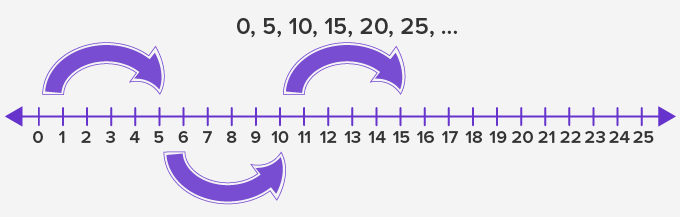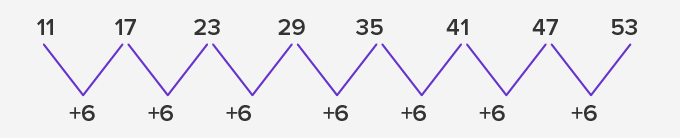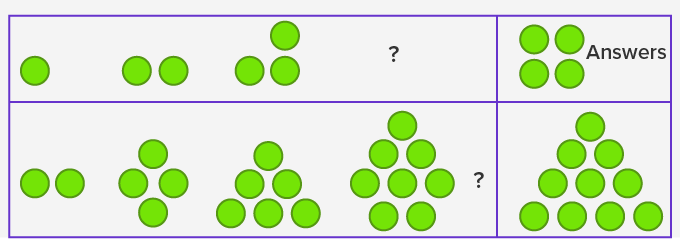# Number Patterns - Definition with Examples

The Complete K-5 Math Learning Program Built for Your Child

• 40 Million Kids

Loved by kids and parent worldwide

• 50,000 Schools

Trusted by teachers across schools

• Comprehensive Curriculum

Aligned to Common Core

## Number Pattern

Number pattern is a pattern or sequence in a series of numbers. This pattern generally establishes a common relationship between all numbers.

For example:

0, 5, 10, 15, 20, 25, ...Here, we get the numbers in the pattern by skip counting by 5. Given are the steps to identify a number pattern.

To solve the problems of number pattern, we need first to find the rule being followed in the pattern.

To find out the rule, we need to see the first few numbers in the series.

Try to see the difference between consecutive numbers, it will help us understand the relationship between the numbers.

Example 1:

·         11, 17, 23, 29, 35, 41, 47, 53In this pattern, we see that every term in the sequence has grown or increased by 6 or the difference between any two consecutive numbers is 6. So, we can get the next term by adding 6 to the previous term.

Example 2:

·         18, 15, 12, 9, 6, 3In this number pattern, we can see that every term in the sequence has reduced by 3 or 3 has been subtracted from every number compared to its previous one. So, we can subtract 3 from the previous term to get the next term.

In the above two examples, the number pattern is formed by a common difference in all its terms.

## Patterns with dots

Some problems for the pattern can also involve a pattern of dots, where we need to find out the number and position of the dots in the pattern.

For example:In the given examples, we found out the pattern by finding the dots that were added to the next figure.

 Fun Fact:  Number patterns are not restricted to a few types. They could be ascending, descending, multiples of a certain number, or series of even numbers, odd numbers etc.

Won Numerous Awards & Honors